Prealgebra

# 4.4Add and Subtract Fractions with Common Denominators

Prealgebra4.4 Add and Subtract Fractions with Common Denominators

### Learning Objectives

By the end of this section, you will be able to:
• Add fractions with a common denominator
• Model fraction subtraction
• Subtract fractions with a common denominator

### Be Prepared 4.4

Before you get started, take this readiness quiz.

1. Simplify: $2x+9+3x−4.2x+9+3x−4.$
If you missed this problem, review Example 2.22.
2. Draw a model of the fraction $34.34.$
If you missed this problem, review Example 4.2.
3. Simplify: $3+26.3+26.$
If you missed this problem, review Example 4.48.

How many quarters are pictured? One quarter plus $22$ quarters equals $33$ quarters.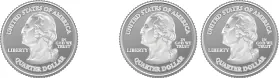Remember, quarters are really fractions of a dollar. Quarters are another way to say fourths. So the picture of the coins shows that

$142434one quarter+two quarters=three quarters142434one quarter+two quarters=three quarters$

Let’s use fraction circles to model the same example, $14+24.14+24.$

 Start with one $1414$ piece.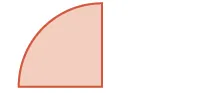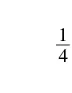Add two more $1414$pieces.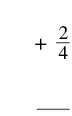The result is $3434$.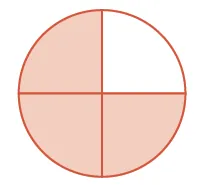So again, we see that

$14+24=3414+24=34$

### Example 4.52

Use a model to find the sum $38+28.38+28.$

### Try It 4.103

Use a model to find each sum. Show a diagram to illustrate your model.

$18+4818+48$

### Try It 4.104

Use a model to find each sum. Show a diagram to illustrate your model.

$16+4616+46$

### Add Fractions with a Common Denominator

Example 4.52 shows that to add the same-size pieces—meaning that the fractions have the same denominator—we just add the number of pieces.

If $a,b,andca,b,andc$ are numbers where $c≠0,c≠0,$ then

$ac+bc=a+bcac+bc=a+bc$

To add fractions with a common denominators, add the numerators and place the sum over the common denominator.

### Example 4.53

Find the sum: $35+15.35+15.$

### Try It 4.105

Find each sum: $36+26.36+26.$

### Try It 4.106

Find each sum: $310+710.310+710.$

### Example 4.54

Find the sum: $x3+23.x3+23.$

### Try It 4.107

Find the sum: $x4+34.x4+34.$

### Try It 4.108

Find the sum: $y8+58.y8+58.$

### Example 4.55

Find the sum: $−9d+3d.−9d+3d.$

### Try It 4.109

Find the sum: $−7d+8d.−7d+8d.$

### Try It 4.110

Find the sum: $−6m+9m.−6m+9m.$

### Example 4.56

Find the sum: $2n11+5n11.2n11+5n11.$

### Try It 4.111

Find the sum: $3p8+6p8.3p8+6p8.$

### Try It 4.112

Find the sum: $2q5+7q5.2q5+7q5.$

### Example 4.57

Find the sum: $−312+(−512).−312+(−512).$

### Try It 4.113

Find each sum: $−415+(−615).−415+(−615).$

### Try It 4.114

Find each sum: $−521+(−921).−521+(−921).$

### Model Fraction Subtraction

Subtracting two fractions with common denominators is much like adding fractions. Think of a pizza that was cut into $1212$ slices. Suppose five pieces are eaten for dinner. This means that, after dinner, there are seven pieces (or $712712$ of the pizza) left in the box. If Leonardo eats $22$ of these remaining pieces (or $212212$ of the pizza), how much is left? There would be $55$ pieces left (or $512512$ of the pizza).

$712−212=512712−212=512$

Let’s use fraction circles to model the same example, $712−212.712−212.$

Start with seven $112112$ pieces. Take away two $112112$ pieces. How many twelfths are left?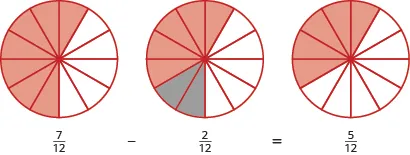Again, we have five twelfths, $512.512.$

### Manipulative Mathematics

Doing the Manipulative Mathematics activity "Model Fraction Subtraction" will help you develop a better understanding of subtracting fractions.

### Example 4.58

Use fraction circles to find the difference: $45−15.45−15.$

### Try It 4.115

Use a model to find each difference. Show a diagram to illustrate your model.

$78−4878−48$

### Try It 4.116

Use a model to find each difference. Show a diagram to illustrate your model.

$56−4656−46$

### Subtract Fractions with a Common Denominator

We subtract fractions with a common denominator in much the same way as we add fractions with a common denominator.

### Fraction Subtraction

If $a,b,andca,b,andc$ are numbers where $c≠0,c≠0,$ then

$ac−bc=a−bcac−bc=a−bc$

To subtract fractions with a common denominators, we subtract the numerators and place the difference over the common denominator.

### Example 4.59

Find the difference: $2324−1424.2324−1424.$

### Try It 4.117

Find the difference: $1928−728.1928−728.$

### Try It 4.118

Find the difference: $2732−1132.2732−1132.$

### Example 4.60

Find the difference: $y6−16.y6−16.$

### Try It 4.119

Find the difference: $x7−27.x7−27.$

### Try It 4.120

Find the difference: $y14−1314.y14−1314.$

### Example 4.61

Find the difference: $−10x−4x.−10x−4x.$

### Try It 4.121

Find the difference: $−9x−7x.−9x−7x.$

### Try It 4.122

Find the difference: $−17a−5a.−17a−5a.$

Now lets do an example that involves both addition and subtraction.

### Example 4.62

Simplify: $38+(−58)−18.38+(−58)−18.$

### Try It 4.123

Simplify: $25+(−45)−35.25+(−45)−35.$

### Try It 4.124

Simplify: $59+(−49)−79.59+(−49)−79.$

### Section 4.4 Exercises

#### Practice Makes Perfect

In the following exercises, use a model to add the fractions. Show a diagram to illustrate your model.

254.

$2 5 + 1 5 2 5 + 1 5$

255.

$3 10 + 4 10 3 10 + 4 10$

256.

$1 6 + 3 6 1 6 + 3 6$

257.

$3 8 + 3 8 3 8 + 3 8$

Add Fractions with a Common Denominator

In the following exercises, find each sum.

258.

$4 9 + 1 9 4 9 + 1 9$

259.

$2 9 + 5 9 2 9 + 5 9$

260.

$6 13 + 7 13 6 13 + 7 13$

261.

$9 15 + 7 15 9 15 + 7 15$

262.

$x 4 + 3 4 x 4 + 3 4$

263.

$y 3 + 2 3 y 3 + 2 3$

264.

$7 p + 9 p 7 p + 9 p$

265.

$8 q + 6 q 8 q + 6 q$

266.

$8 b 9 + 3 b 9 8 b 9 + 3 b 9$

267.

$5 a 7 + 4 a 7 5 a 7 + 4 a 7$

268.

$−12 y 8 + 3 y 8 −12 y 8 + 3 y 8$

269.

$−11 x 5 + 7 x 5 −11 x 5 + 7 x 5$

270.

$− 1 8 + ( − 3 8 ) − 1 8 + ( − 3 8 )$

271.

$− 1 8 + ( − 5 8 ) − 1 8 + ( − 5 8 )$

272.

$− 3 16 + ( − 7 16 ) − 3 16 + ( − 7 16 )$

273.

$− 5 16 + ( − 9 16 ) − 5 16 + ( − 9 16 )$

274.

$− 8 17 + 15 17 − 8 17 + 15 17$

275.

$− 9 19 + 17 19 − 9 19 + 17 19$

276.

$6 13 + ( − 10 13 ) + ( − 12 13 ) 6 13 + ( − 10 13 ) + ( − 12 13 )$

277.

$5 12 + ( − 7 12 ) + ( − 11 12 ) 5 12 + ( − 7 12 ) + ( − 11 12 )$

Model Fraction Subtraction

In the following exercises, use a model to subtract the fractions. Show a diagram to illustrate your model.

278.

$5 8 − 2 8 5 8 − 2 8$

279.

$5 6 − 2 6 5 6 − 2 6$

Subtract Fractions with a Common Denominator

In the following exercises, find the difference.

280.

$4 5 − 1 5 4 5 − 1 5$

281.

$4 5 − 3 5 4 5 − 3 5$

282.

$11 15 − 7 15 11 15 − 7 15$

283.

$9 13 − 4 13 9 13 − 4 13$

284.

$11 12 − 5 12 11 12 − 5 12$

285.

$7 12 − 5 12 7 12 − 5 12$

286.

$4 21 − 19 21 4 21 − 19 21$

287.

$− 8 9 − 16 9 − 8 9 − 16 9$

288.

$y 17 − 9 17 y 17 − 9 17$

289.

$x 19 − 8 19 x 19 − 8 19$

290.

$5 y 8 − 7 8 5 y 8 − 7 8$

291.

$11 z 13 − 8 13 11 z 13 − 8 13$

292.

$− 8 d − 3 d − 8 d − 3 d$

293.

$− 7 c − 7 c − 7 c − 7 c$

294.

$− 23 u − 15 u − 23 u − 15 u$

295.

$− 29 v − 26 v − 29 v − 26 v$

296.

$6 c 7 − 5 c 7 6 c 7 − 5 c 7$

297.

$12 d 11 − 9 d 11 12 d 11 − 9 d 11$

298.

$−4 r 13 − 5 r 13 −4 r 13 − 5 r 13$

299.

$−7 s 3 − 7 s 3 −7 s 3 − 7 s 3$

300.

$− 3 5 − ( − 4 5 ) − 3 5 − ( − 4 5 )$

301.

$− 3 7 − ( − 5 7 ) − 3 7 − ( − 5 7 )$

302.

$− 7 9 − ( − 5 9 ) − 7 9 − ( − 5 9 )$

303.

$− 8 11 − ( − 5 11 ) − 8 11 − ( − 5 11 )$

Mixed Practice

In the following exercises, perform the indicated operation and write your answers in simplified form.

304.

$− 5 18 · 9 10 − 5 18 · 9 10$

305.

$− 3 14 · 7 12 − 3 14 · 7 12$

306.

$n 5 − 4 5 n 5 − 4 5$

307.

$6 11 − s 11 6 11 − s 11$

308.

$− 7 24 + 2 24 − 7 24 + 2 24$

309.

$− 5 18 + 1 18 − 5 18 + 1 18$

310.

$8 15 ÷ 12 5 8 15 ÷ 12 5$

311.

$7 12 ÷ 9 28 7 12 ÷ 9 28$

#### Everyday Math

312.

Trail Mix Jacob is mixing together nuts and raisins to make trail mix. He has $610610$ of a pound of nuts and $310310$ of a pound of raisins. How much trail mix can he make?

313.

Baking Janet needs $5858$ of a cup of flour for a recipe she is making. She only has $3838$ of a cup of flour and will ask to borrow the rest from her next-door neighbor. How much flour does she have to borrow?

#### Writing Exercises

314.

Greg dropped his case of drill bits and three of the bits fell out. The case has slots for the drill bits, and the slots are arranged in order from smallest to largest. Greg needs to put the bits that fell out back in the case in the empty slots. Where do the three bits go? Explain how you know.
Bits in case: $116116$, $1818$, ___, ___, $516516$, $3838$, ___, $1212$, $916916$, $5858$.
Bits that fell out: $716716$, $316316$, $1414$.

315.

After a party, Lupe has $512512$ of a cheese pizza, $412412$ of a pepperoni pizza, and $412412$ of a veggie pizza left. Will all the slices fit into $11$ pizza box? Explain your reasoning.

#### Self Check

After completing the exercises, use this checklist to evaluate your mastery of the objectives of this section.

On a scale of 1–10, how would you rate your mastery of this section in light of your responses on the checklist? How can you improve this?

Order a print copy

As an Amazon Associate we earn from qualifying purchases.